×

Search anything:

# Pancreas Segmentation using Attention U-Net [with code]

#### Deep LearningGet this book -> Problems on Array: For Interviews and Competitive Programming

In this article, we are going to walk through a smart way to reduce the computation power needed for an biomedical image analysis which is attention and its use in computer vision tasks. We solve the problem of Pancreas Segmentation using Attention U-Net and implement it in Python using TensorFlow.

This is a strong project for Deep Learning portfolio.

1. Introduction and problem statement
2. What is attention?
3. How we modify the basic U-Net model?
4. Methodology
• Attention Gates in U-Net Model
5. Datasets used
6. Results
7. Code for Attention U-net

## Introduction and problem statement

Pancreas is an organ in human body near the abdomen which produce enzymes to help with digestion and produce enzymes to control the amount of sugar in your blood. There are several problems with pancreas which need to be treated and Deep Learning can help with the process.

Pancreas Segmentation is the process of segmentation that is identifying the exact location and boundary of pancreas in an abdomen scan. Such scans can be unclear and difficult to accurately identify exact structure of pancreas. The exact structure is important to identify abnormal growth and do correct treatment.

Nowadays, image segmentation technique achieve a very good results specially in medical images field and in the same time manual labeling of large amounts of medical images is a tedious and error-prone task. So the growing need to fast and accurate techniques is to increase clinical work flow efficiency and decrease the time for it.

With convolution neural networks(CNNs), near-radiologist level performance can be achieved in automated medical image analysis tasks including cardiac MR segmentation and cancerous lung nodule detection. U-Net, DeepLab, Mask R-CNN and V-Net are a very common CNN architectures that achieve a very good results for image segmentation, but these architectures rely on multi-stage cascaded CNNs when the target organs show large inter-patient variation in terms of shape and size (like searching the pancreas) which segmentation is necessary nowadays for observing lesions, analyzing anatomical structures, and predicting patient prognosis as a good segmentation of the pancreas can lead to:

• Accurate diagnosis: Segmentation of the pancreas can provide clinicians with a more accurate picture of the size, shape, and location of the pancreas. This information is crucial for the diagnosis of pancreatic diseases, such as pancreatic cancer, pancreatitis, and cysts.
• Treatment planning: Segmentation of the pancreas can help in planning for surgical or radiation therapy for pancreatic cancer. Accurate segmentation helps in determining the precise location and extent of the tumor, which is essential for treatment planning.
• Drug delivery: Pancreatic drug delivery can be challenging due to the complex anatomy of the pancreas. Accurate segmentation of the pancreas can help in identifying the optimal delivery site and improve the effectiveness of drug therapy.
• And finally in Research: Pancreatic segmentation is also crucial for research purposes, particularly in the development and testing of new diagnostic and therapeutic methods for pancreatic diseases.

Cascaded frameworks extract a region of interest (ROI) and make dense predictions on that particular ROI. So, this approach leads to excessive and redundant use of computational resources and model parameters; for instance, similar low-level features are repeatedly extracted by all models within the cascade.
And, As we will see that introducing attention (attention gates) to the U-net will show a progress on the results.

## What is attention?

Attention is a way to focus and highlight the important region of the image and focus to less extend to the other parts which i prefer to call distractors, and to achieve this we have to ways as following :

Hard attention--> which achieved by cropping the important part the pass it to the CNN network, meaning that instead of introducing the whole image to the network, we will introduce the important parts only.

So this operation need Reinforcement learning to learn as it is not a differential operation and we can't use back-propagation process.
and also it is all or none process, either it pay attention or not, nothing in between.

Soft attention --> which can be achieved by giving weight to different parts of the image, higher weights to relevant parts and lower weights to irrelevant parts and this weight can be trained during the model training process. So back-propagation can be used.

In this article, we try to introduce this mechanism to the U-net model as proposal of Attention Gates (AG) to the model and see the effect of this introduction.

## How we modify the basic U-Net model?

In this approach we will introduce additional blocks called Attention Gates(AG) to a standard U-Net architecture (Attention U-Net) and apply it to medical images. we decide to try this method to 2 of famous and difficult datasets CT-82 and multi-class abdominal CT-150 which consider a challenge as low tissue contrast and large variability in organ shape and size.But achieving a promising results.

As our new gate focus on target structures of varying shapes and sizes. Models trained with AGs implicitly learn to suppress irrelevant regions in an input image while highlighting salient features

## Methodology

Fully convolution neural network such as U-net have been shown to achieve robust and accurate performance in various tasks including cardiac MR, brain tumors and abdominal CT image segmentation tasks. So introducing this AG will improve the model.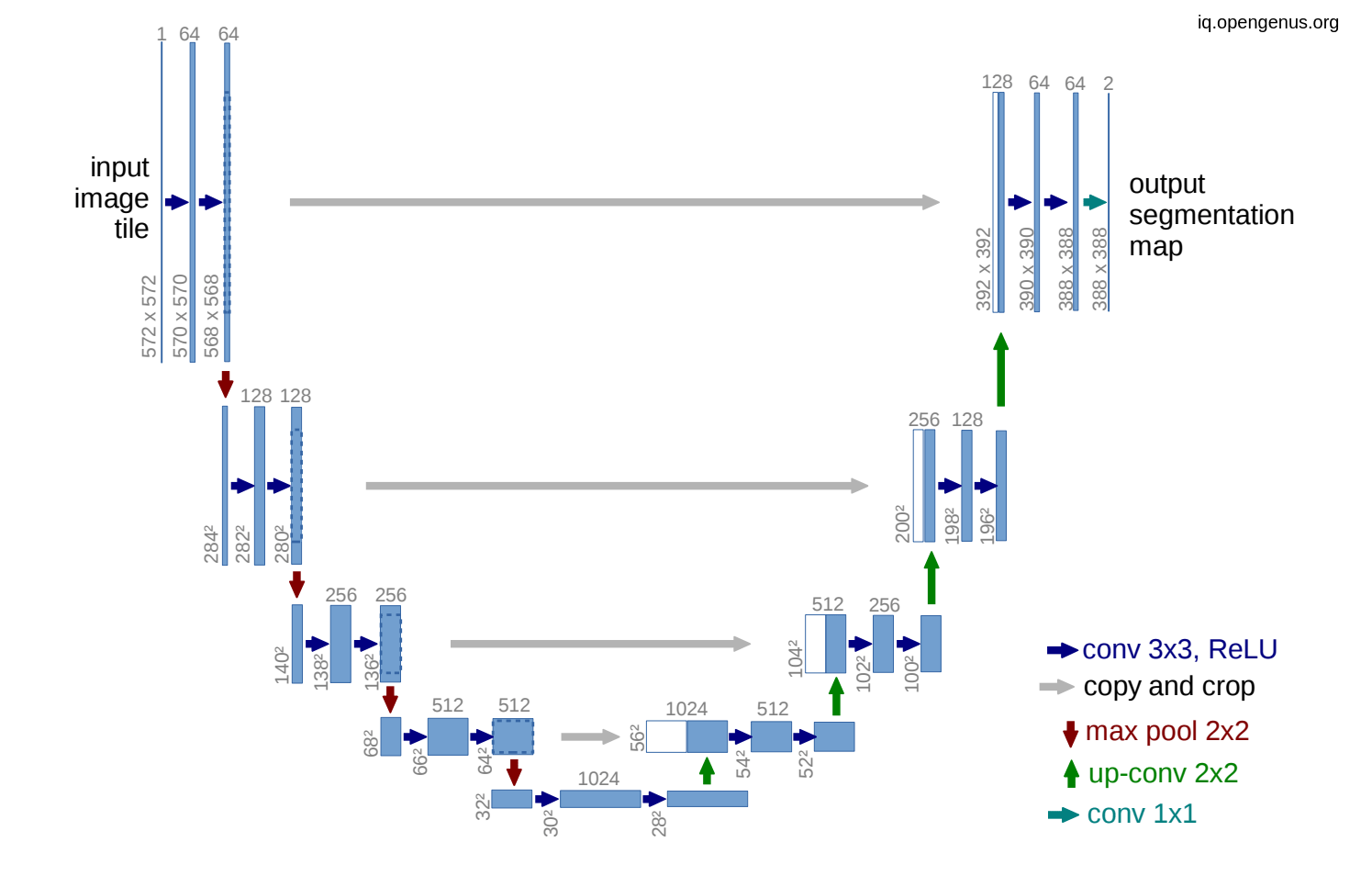In the U-net model we use skip connection in order to introduce spatial information from down-sampling network(encoder) to up-sampling network (decoder) but this skip connection bring also poor feature representation as in come from initial layers. So adding this attention gates will suppress activation on irrelevant.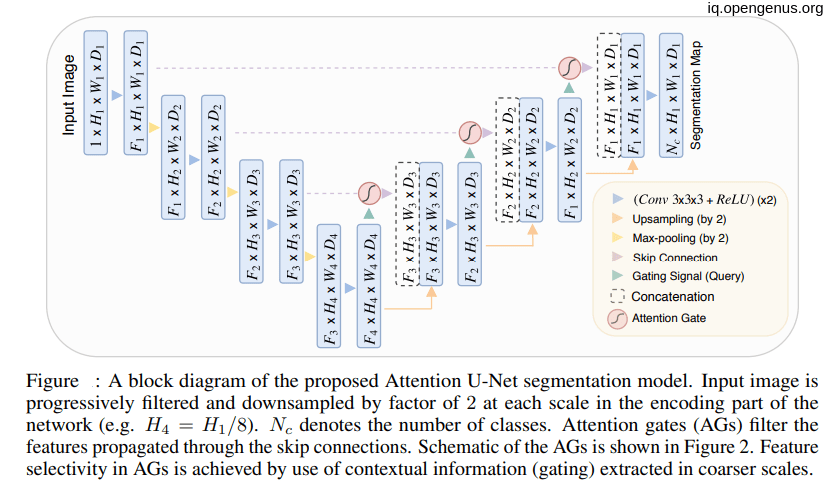.

### Attention Gates in U-Net Model

As we see in the above figure that the attention gates receive information from two inputs one come from the next lowest layer of the network(g) and the other come from skip connections(x).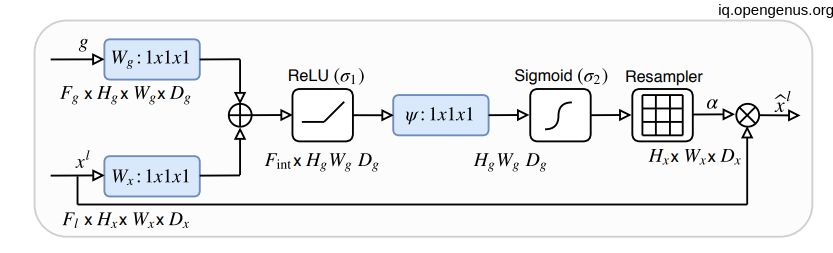Let's see what is happening-->

As we know that x is coming from one layer upper so it will be have the double of H and W dimension So before adding them together we had to convolute the X with stride of 2 in order to equal the dimensionality. then doing the element-wise addition then relu activation then convolute with only one (1,1) filter with Sigmoid activation function then up-sampling the result (which is nothing except the weights of attention) with the shape of x then element-wise multiplication.

Example of AG: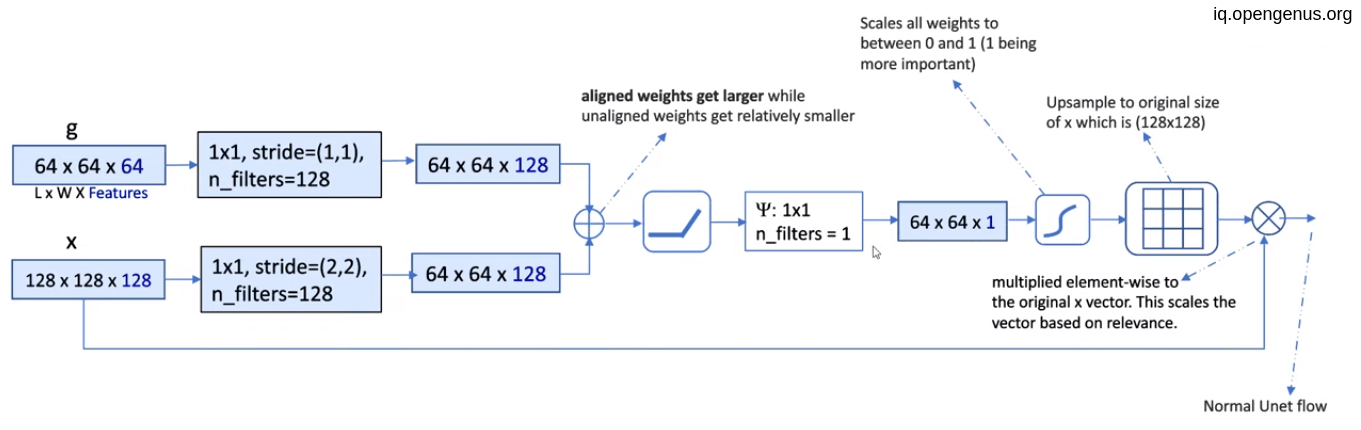Assuming that g = (64,64,64) and x = (128,128,128)

1. convolute g with 128 filters of (1,1) with stride (1,1), resulting (64,64,128)
2. convolute x with 128 filters of (1,1) with stride (2,2) to equal the g shape, resulting (64,64,128)
3. Element-wise addition of two tensors.
4. Relu activation to the result of addition.
5. then convolute the result with only one filter of (1,1) resulting (64,64,1) which is the weights for attention.
6. Up-sampling the weight to equal to x to give a weight to each pixel.
7. Then element-wise multiplication of each pixel with its weight.
8. Then concatenate the result with the corresponding result from Up-sampling process in the U-net model and go on.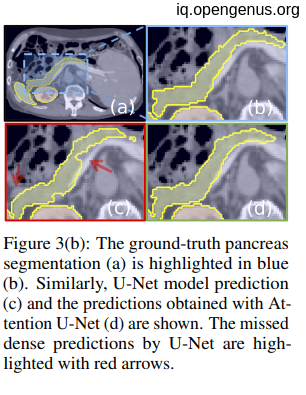## Datasets used

1. 150 abdominal 3D CT scans acquired from patients diagnosed with gastric cancer (CT-150).
2. (CT-82) consists of 82 contrast enhanced 3D CT scans with pancreas manual annotations performed slice-by-slice.

## Results

the following tables showing that the introduction of the attention to the U-net model have a good impact on the model: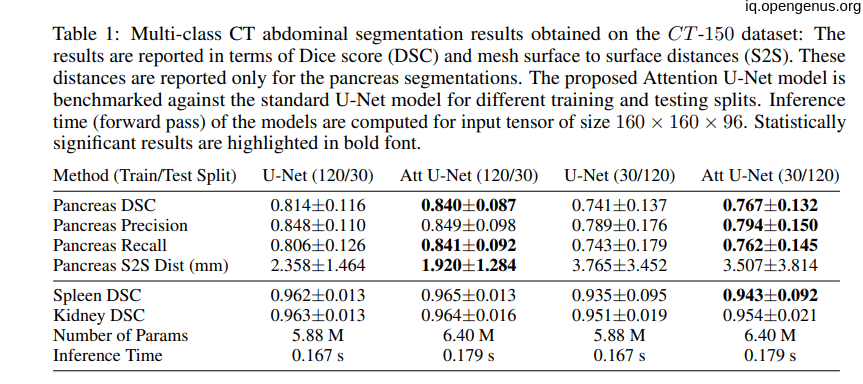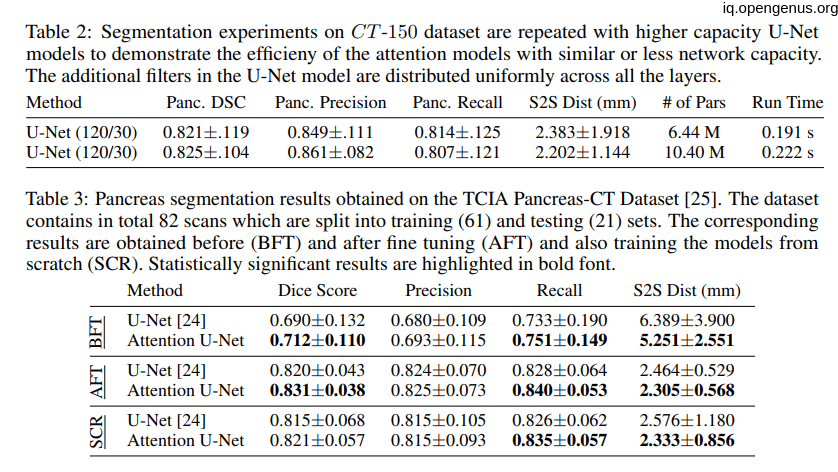Comparison to State-of-the-Art:
the next image showing the State-of-the-Art technique on CT-150 and CT-82 datasets.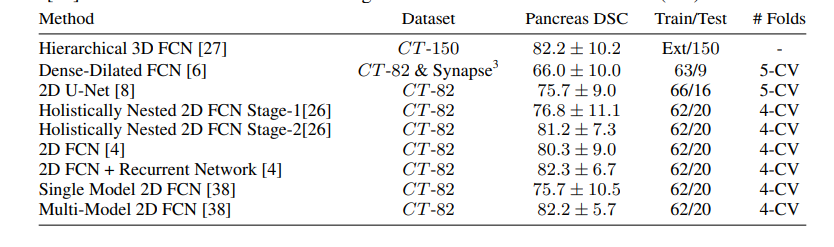the Attention U-Net for a better comparison, which achieved 81.48 ± 6.23 DSC for pancreas labels.

## Code for Attention U-net

Following is the complete Python code using TensorFlow to build our Attention U-Net model for Pancreas Segmentation:

``````import tensorflow as tf
import tensorflow.keras.backend as K

#definig the convolution block that consist of 2 conv layers.

def conv_block(input,no_filter,f_size,BN = False,drop_out = 0):
'''
arg:
input--> the input to the block which is the output of the previous block
no_filter --> number of filters used in the conv layers
f_size --> size of the kernel
BN --> batch normalization layer (bolean)
drop_out --> dropout ratio for regularization
'''
if BN :
x = tf.keras.layers.BatchNormalization()(x)
if BN :
x = tf.keras.layers.BatchNormalization()(x)
if drop_out > 0 :
x = tf.keras.layers.Dropout(drop_out)(x)
return x

``````

lambda function for repeating the result from AG:

``````#lambda function for repeating the result from AG
def repeat_elem(tensor, rep):

return tf.keras.layers.Lambda(lambda x, repnum: K.repeat_elements(x, repnum, axis=3),
arguments={'repnum': rep})(tensor)
``````

Defining the attention blocks that take 2 input and dimentionality:

``````#defining the attention blocks that take 2 input and dimentionality

def att_block(x,g, desired_dimensionality):
'''
x-->> the input from skip connection
g-->> the input from next lower
'''
x_shape = x.shape
g_shape = g.shape
#strides for xl should be 2 to equal the shapes before addition
xg = tf.keras.layers.Activation("relu")(xg)
xg_shape = xg.shape
xg = tf.keras.layers.UpSampling2D((x_shape//xg_shape,x_shape//xg_shape))(xg)
#repetion for equal the dimensionality
xg = repeat_elem(xg, x_shape[-1])
output = tf.keras.layers.Multiply()([xg,x])
return output
``````

### Our final model function

``````def att_model(no_filter,input_shape=(224,224,3) ):
#down-sampling process
inputs = tf.keras.layers.Input(input_shape, dtype=tf.float32)
x1= conv_block(inputs,no_filter,(3,3),BN = True,drop_out = 0)
pool1= tf.keras.layers.MaxPooling2D(2,2)(x1)

x2= conv_block(pool1,2*no_filter,(3,3),BN = True,drop_out = 0)
pool2= tf.keras.layers.MaxPooling2D(2,2)(x2)

x3= conv_block(pool2,4*no_filter,(3,3),BN = True,drop_out = 0)
pool3= tf.keras.layers.MaxPooling2D(2,2)(x3)

x4= conv_block(pool3,8*no_filter,(3,3),BN = True,drop_out = 0)
pool4= tf.keras.layers.MaxPooling2D(2,2)(x4)

#bottle-neck
x5= conv_block(pool4,16*no_filter,(3,3),BN = True,drop_out = 0)

#up-sampling layers

x6= att_block(x4,x5, no_filter*2)
u6= tf.keras.layers.UpSampling2D(2)(x5)
concate1 = tf.keras.layers.Concatenate()([x6,u6])
conv6 = conv_block(concate1,8*no_filter,(3,3),BN = True,drop_out = 0)

x7= att_block(x3,conv6, no_filter*2)
u7= tf.keras.layers.UpSampling2D(2)(conv6)
concate2 = tf.keras.layers.Concatenate()([x7,u7])
conv7 = conv_block(concate2,4*no_filter,(3,3),BN = True,drop_out = 0)

x8= att_block(x2,conv7, no_filter*2)
u8= tf.keras.layers.UpSampling2D(2)(conv7)
concate3 = tf.keras.layers.Concatenate()([x8,u8])
conv8 = conv_block(concate3,2*no_filter,(3,3),BN = True,drop_out = 0)

x9= att_block(x1,conv8, no_filter*2)
u9= tf.keras.layers.UpSampling2D(2)(conv8)
concate4 = tf.keras.layers.Concatenate()([x9,u9])
conv8 = conv_block(concate4,no_filter,(3,3),BN = True,drop_out = 0)

conv_final = tf.keras.layers.Conv2D(1, kernel_size=(1,1))(conv8)
conv_final =tf.keras.layers.BatchNormalization(axis=3)(conv_final)
conv_final = tf.keras.layers.Activation('sigmoid')(conv_final)

return tf.keras.Model(inputs,conv_final)

``````

Defining loss function suitable for semantic segmentation as Dice score.

``````import tensorflow.keras.backend as K

def dice_loss(y_true, y_pred, smooth=1):
intersection = K.sum(K.abs(y_true * y_pred), axis=-1)
union = K.sum(y_true, axis=-1) + K.sum(y_pred, axis=-1)
dice = (2. * intersection + smooth) / (union + smooth)
loss = 1. - K.mean(dice)
return loss

``````

Instance of model with Adam optimizer.

``````model = att_model(32,input_shape=(224,224,3) )
loss=dice_loss)
``````

With this article at OpenGenus, you must have the complete idea of Pancreas Segmentation using Attention U-Net.